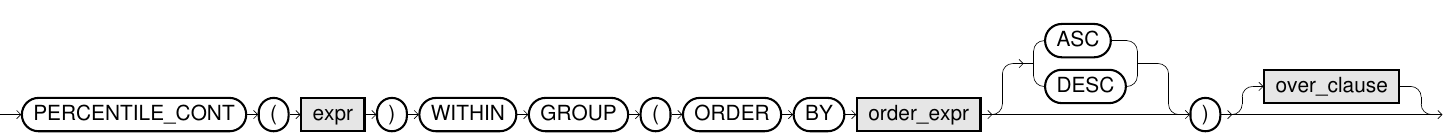# PERCENTILE_CONT

## Purpose

PERCENTILE_CONT is an inverse distribution function and expects as an input parameter a percentile value and a sorting specification which defines the rank of each element within a group. The functions return the percentile of the sort order as per the following example - in the case of percentile 0.7 and 100 values, the 70th value is returned.

If the percentile cannot be assigned exactly to an element, then the linear interpolation between the two nearest values is returned. For example, in the case of percentile 0.71 and ten values, the interpolation between the 7th and 8th value.

## Syntax

percentile_cont::=## Usage Notes

• NULL values are ignored for the computation.
• The expr must be a numeric value between 0 and 1. The expr must be constant within a partition. This implies that expr may depend on the partitioning attribute, so that it may vary in different partitions but must remain constant for all rows within a partition. Exasol returns an error when this condition is not satisfied.
• The order_clause is necessary for PERCENTILE_CONT function.
• The over_clause must not contain an order_clause and must not contain a window_frame_clause.
• For information about the over_clause and analytic functions in general, refer to the Analytic Functions section.
• For additional information, also refer to the inverse distribution functions PERCENTILE_DISC and MEDIAN.

## Example

### Analytic Function

``SELECT     id, department, current_salary,     PERCENTILE_CONT(0.7) WITHIN GROUP (ORDER BY current_salary)         OVER (PARTITION BY department) PERCENTILE_CONT FROM employee_table ORDER BY department, current_salary;``
###### Result
 ID DEPARTMENT CURRENT_SALARY PERCENTILE_CONT 2001 ACCOUNTS 55000 78000 2002 ACCOUNTS 65000 78000 2004 ACCOUNTS 70000 78000 2003 ACCOUNTS 80000 78000 2005 ACCOUNTS 80000 78000 1001 HR 55000 72000 1002 HR 70000 72000 1004 HR 70000 72000 1003 HR 90000 72000